# Detailed Explanation of Deep Neural Network, Multilayer Perceptron, and Deep Q-NetworkHumans are forever inspired by nature’s wonders. Whether it was SB Talpade creating the first unmanned aircraft back in 1895, thanks to birds, or MIT researchers building a robotic cheetah in the hopes that it may lead to new types of transport, nature continues to galvanize tech innovations. But perhaps one of the most exciting is neural networks, which mimic the way the human brain works.

With first proposals dating back to the late 1800s, neural nets have come a long way and can do a lot of the things the human brain can. The commercial and scientific applications of this technology have far exceeded expectations and continue to grow exponentially.

This article will examine three of the latest technologies that are possible thanks to neural networks.

## Deep Neural Network

A deep neural network (DNN), popularly known as deep learning, is a subset of machine learning (ML). It’s an attempt by scientists and engineers to mimic the complex neural pathways of a brain, giving lifeless machines some ‘thinking’ capabilities of a human mind.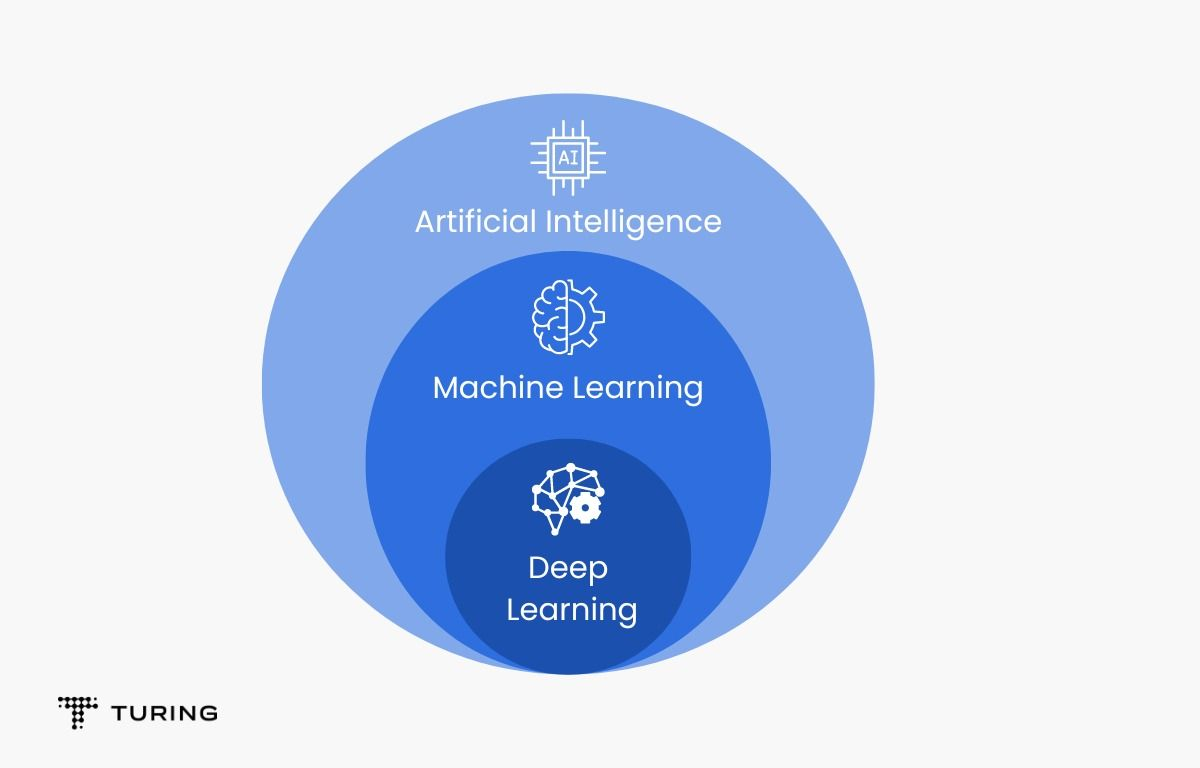### Why do we need deep neural networks?

The applications of machine learning are endless but it fails to perform in certain areas where the human mind can easily outperform any machine. Case in point: it’s very hard for a machine to distinguish between male and female faces, voices, guess their age, etc. ML algorithms seem to poorly perform on such unstructured data, which ultimately led to the creation of deep learning.

Deep learning has applications across various fields like natural language processing, visual recognition, language translation, healthcare, virtual assistants, etc.

### Important terms

The brain is a dense mesh of biological neurons with just one function at its core: learn and adapt from past experiences. A DNN consists of layers and layers of interconnected nodes similar to the neurons in the brain. These nodes pass data based on the signal received, forming a network that learns by receiving feedback from a mathematical function that optimizes it with every iteration.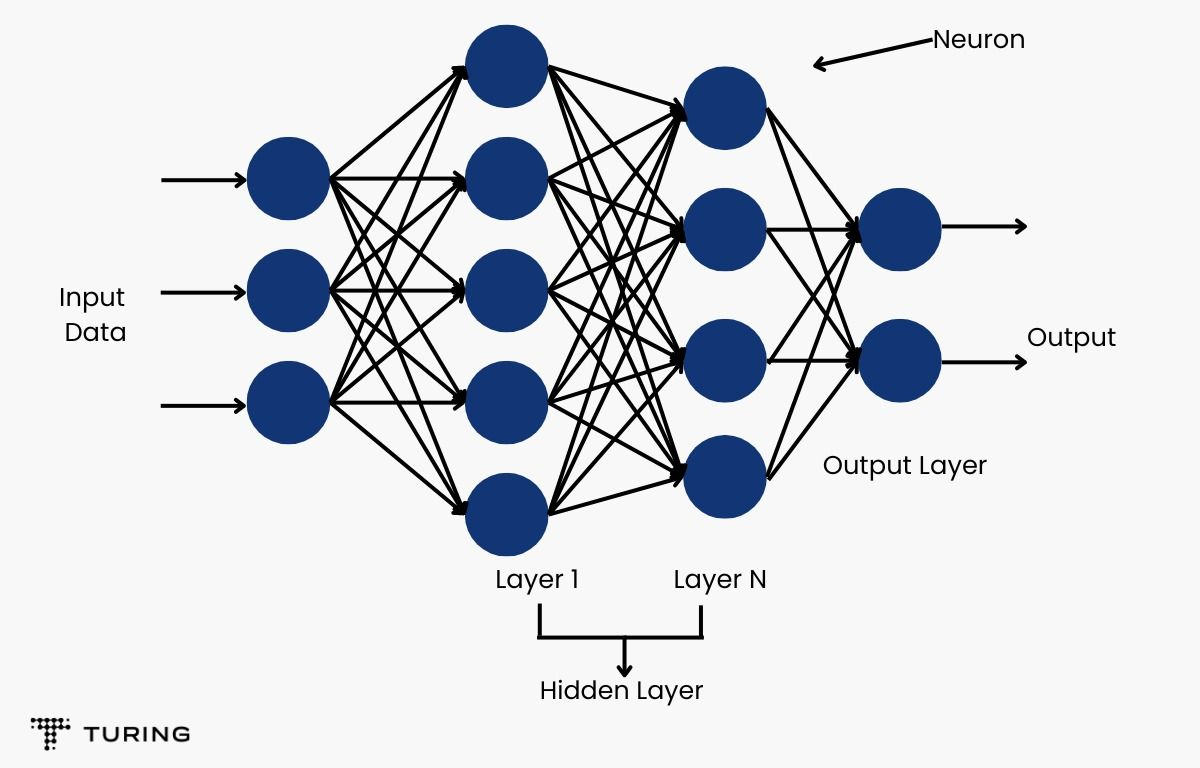The image above shows how the first layers receive data, do the necessary mathematical calculations, and then feed the output data onto the next layer, and so on.

Let’s break down the individual concepts of a deep neural network.

1. Neuron (or nodes): Neurons are the building blocks of a neural network. Depending on their positioning and hierarchy, they receive input, perform the mathematical calculations associated with them, and output onto the next neuron in line, or as the final output.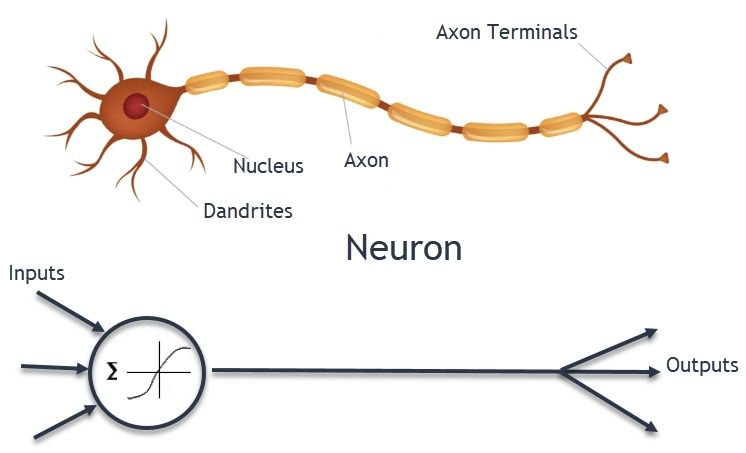Image source: Neural Network Foundations

2. Parameters (or weights): Every input into neurons has a ‘weight’ associated with them. Essentially, it’s an arbitrary value that is assigned by the user and is updated with each epoch of the training process. A feature that has a greater correlation to the target variable gets assigned a higher ‘weight’ with each cycle, while the features that are not so significant are assigned a lower one. This is how the machine ranks the features associated with a problem.

3. Bias: The popular straight line equation y = mx + c has (x,y) as the coordinates, m as the slope, and c as the intercept, a constant term. For a line passing through origin, the value for c is zero. Similarly in neural networks, this intercept is known as ‘bias’, a constant term used to balance the output. Without it, the model trains only over the origin (since c becomes 0), which is not how the real world works.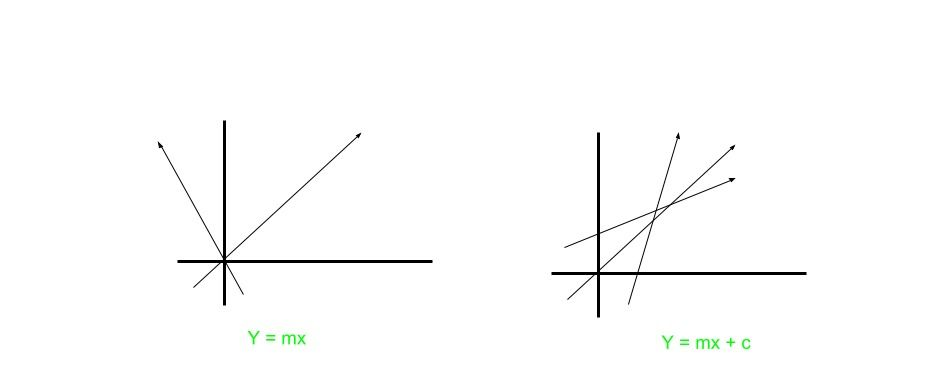Image source:Effect of Bias in Neural Network

4. Activation function: This is the mathematical function present inside every neuron. As the name suggests, it decides whether the neuron gets ‘activated’ and passes the signal onto the next neuron. There are numerous types of activation functions, each with different thresholds. If the calculated value exceeds the threshold, the neuron is not fired, thus remaining unactivated. Some examples of activation functions are Sigmoid, ReLU, Softmax, Tanh, etc.

It’s easy to differentiate between the various parts of a neuron. Here’s a visual aid: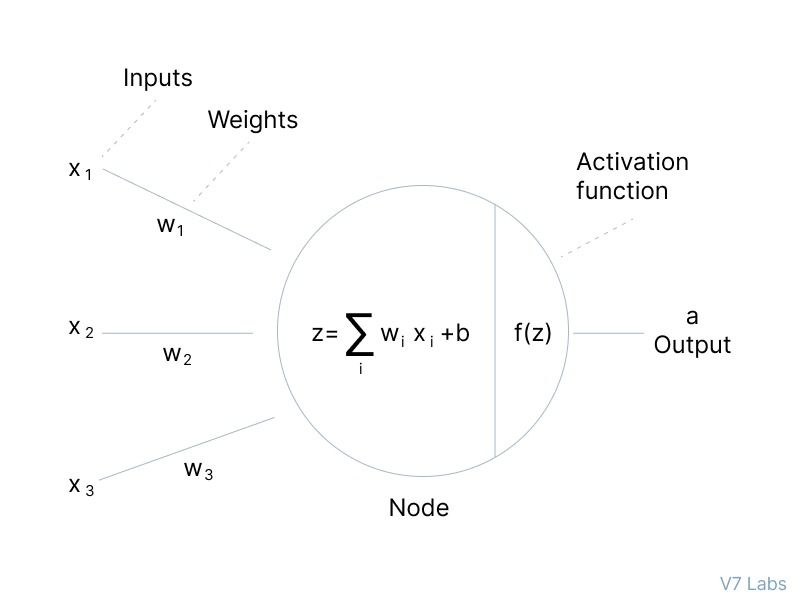Image source

## Multilayer Perceptron

A single neuron hardly makes a dent in the face of a complex calculation. What is needed is a hierarchical mesh of neurons. Multilayer perceptron (MLP) is a complex structure that helps a machine learn a much more sophisticated decision boundary. It has applications in stock price prediction, image classification, spam detection, sentiment analysis, data compression, etc.

A multilayer perceptron has three segments:

• Input layer, where data is fed into the network.
• Hidden layer, where all the mathematical calculations are done using weights, biases, and the data.
• Output layer, where meaningful results are obtained from.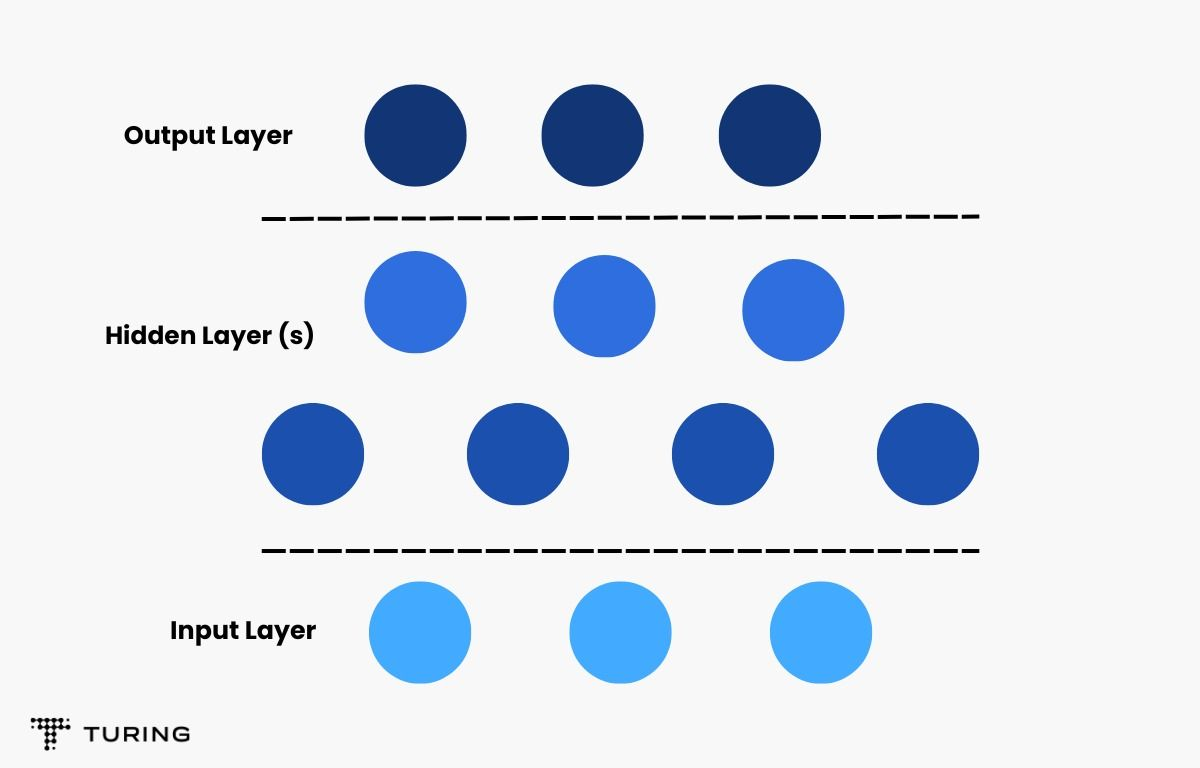MLP uses something called the feedforward algorithm, i.e, the data moves in a single dimension. It starts from the input layer, moves through the hidden layers, if present, and on to the output layer.

The raw information is fed into the input layer where all the data points are multiplied with their respective assigned weights and biases added. This is then subjected to an activation function and the resulting linear combination is relayed onto the next layer.
This process occurs through the input, hidden, and output layers.

But the learning process doesn’t end here.

Weights are randomly initialized by the user. If all the MLP did was add biases and multiply weights to the input, the machine would not be able to learn and customize the weights for optimal performance. This is where backpropagation comes in.

### Backpropagation

Backpropagation is the method of fine-tuning the weights in a neural net to reach an optimal solution to problems.

A loss function is used to measure the error rate at the end of every epoch. After the first iteration, the gradient of the mean squared error across every input-output pair is calculated. The weights of the first hidden layer are replaced by this value of the gradient obtained and the whole process is repeated till the convergence threshold is achieved.Image source: Multilayer Perceptron Explained with Real-Life Example and Python Code: Sentiment Analysis

## Deep Q-Network

The neural networks utilizing deep Q-learning are known as deep Q-network (DQN). To understand deep Q-learning, you need to be familiar with Q-learning.

Q-learning is a form of reinforcement learning. An agent (a type of a bot) is taught to work optimally in an environment by continually rewarding it for specific tasks that you want to achieve in that particular environment.

Q-Learning is best understood with an example. Reinforcement learning is widely used in video games, so here’s an easy example: let’s suppose you’re developing a basketball game and want to add a game mode where the player can play against a bot for practice. In this scenario, the bot is the ‘agent’, the basketball court is the ‘environment’, the ‘state’ is the bot’s ability to throw the ball through the hoop, and throwing the ball, passing to other players in its team, dribbling, tackling other players, etc. are the ‘actions’. Scoring a point after a basket is the ‘reward’.

Something called the Markov decision process (MDP) helps the bot select the best action to perform at a particular state to get the maximum reward possible. This is how an agent is trained using reinforcement learning.

The Q-learning algorithm is simple as the flowchart below shows.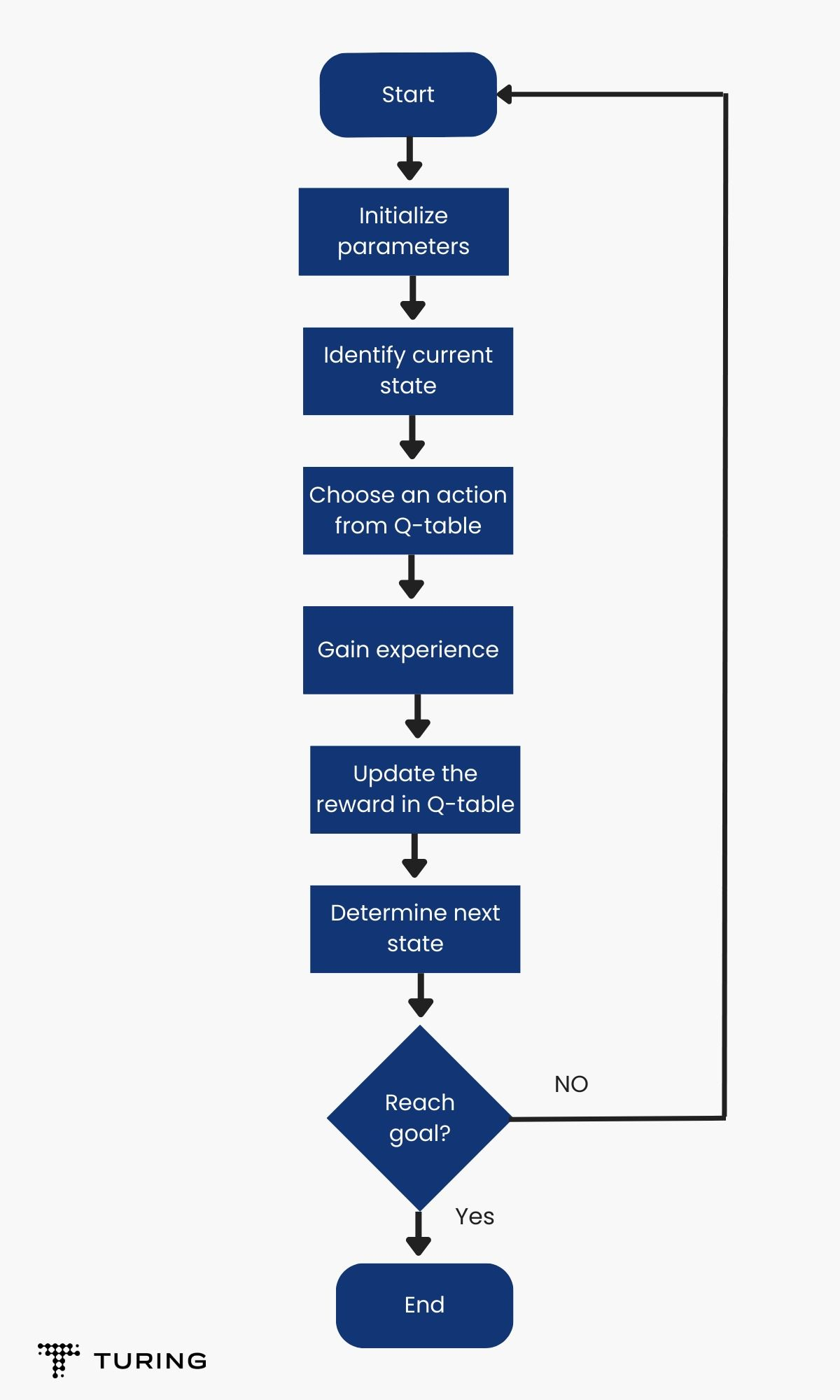### Q-table

The only term you may probably have a slightly hard time understanding from the flowchart above is the Q-table. A Q(s, a) function is used to map the states and actions of the agent and is recorded in a Q-table. Thus, after running the algorithm for some time, you get a Q-table with all the possible ‘state’ and ‘action’ combinations for that particular environment.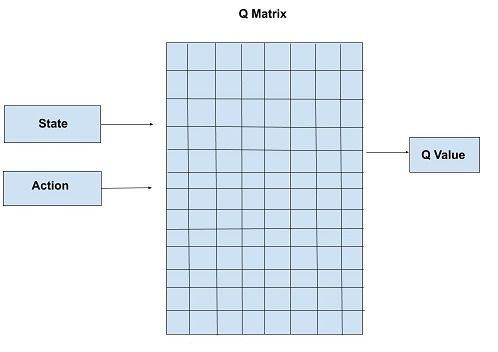The working agent can then refer to this table during later runs to maximize the reward for any given state. Note that this approach is only feasible and limited to small environments with limited action and state pairs.

To combat this limitation, deep neural networks are used alongside the Q-learning algorithm to approximate the values of the Q-table.

### Deep Q-learning

With deep learning and neural networks, you can approximate the Q-table values much faster. The reason approximate values do not affect an agent’s performance is that only the relative values in the Q-table have importance.

The process begins by feeding the initial state into the neural net. The Q-values of all the possible actions are the outputs.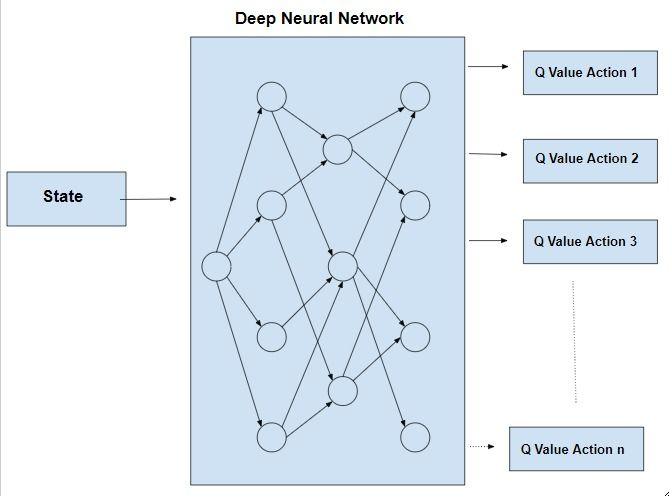Image source:Deep Q-Learning

The integration of neural nets with Q-learning already has numerous applications in up-and-coming fields like self-driving cars, industry automation, financial trading, NLP, medical diagnosis, gaming, etc.

The technologies discussed in this article are still works in progress. Though deep learning is slightly older than the other two, all three continue to be implemented in new ways every now and then. One can argue that deep neural network is really an umbrella term and that multilayer perceptron and deep Q-network are more of a subset or better yet, an application of the former.### Press

What's up with Turing? Get the latest news about us here.### Blog